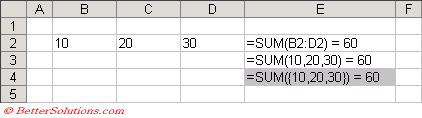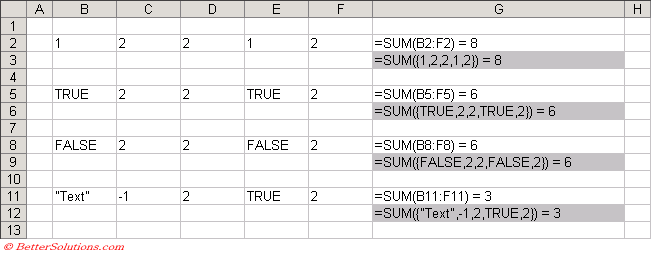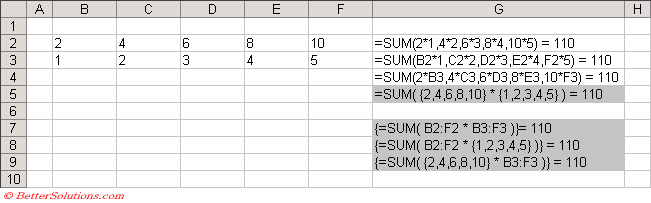# Array Formulas

Also known as CSE Formulas or (Ctrl + Shift + Enter) Formulas.
An array formula is a special type of formula that works specifically with arrays.
An array formula can produce a single result or multiple results where each result is displayed in a separate cell.
An array formula can occupy a single cell or a range of cells.
An array is basically just a collection of data of the same type that can be treated as a single entity. This is held in memory.
For those of you that are not familiar with the concept of an array, it is just a collection of values (in this case typically numbers).
Excel automatically puts curly brackets {} around any array formulas. These brackets cannot be entered manually.

Example 1 - The table below shows how you can use an array constant.Exactly the same result can be obtained by placing the numbers into an array and passing the array (as a whole) to the worksheet function.
Array Constants can contain numbers, logical values (i.e. True or False) or text.
You can use different types of data in the same array constant.

Example 2 - The table below shows more array constants.### Difference with Array Formulas

Example 3 - The table below shows more array constants.All the formulas in column F are equivalent and all return the total 110.
Formulas can work with arrays that are contained in cells, arrays that are constant as well as array formulas.

### Advantages of using Array Formulas

Array formulas guarantee that all the formulas are the same and is a good way to ensure consistency among your formulas.
Using array formulas can speed up the time taken for formulas to be evaluated since the arrays can be stored in memory.
A workbook that contains array formulas will mean that the formulas are more efficient and will use less memory helping to reduce file sizes.
Array formulas help to protect your formulas as you cannot change, clear or move any individual cells that are part of an array block. It is not possible to change the contents of a single cell in an array formula. The only way to change the individual cells to treat the whole block as a single unit and change the whole block at the same time.
You can only delete an array formula by selecting the whole array block first. You cannot delete part of it.
Using an array formula will almost always be quicker than defining a custom function using VBA.
A worksheet that contains array formulas will be harder to understand, meaning that novices are less likely to change the formulas, especially if they do not understand how they work.
Array formulas do make it possible to perform some calculations that would otherwise be impossible using traditional formulas.

### Disadvantages of using Array Formulas

You should try to avoid using a lot of large array formulas in a single workbook. This will reduce your workbook performance as well as making your formulas a nightmare to understand.
To many array formulas will slow down recalculation, saving, opening and closing.
Array formulas use less memory and they can eliminate the need for intermediate formulas, although they can slow the recalculation time of your spreadsheet and make it more complicated.
You cannot use column references (such as "A:A" or "D:D") in your array formulas.
You must always enter them using (Ctrl + Shift + Enter).

### Important

Using a lot of array formulas in one workbook will increase the time taken to re-calculate.
The majority of the calculations that can be performed using array formulas can also be performed using regular formulas. Although there are a few exceptions.
If you accidentally enter an array formula by not pressing (Ctrl + Shift + Enter) then the formula will either return an incorrect value or #VALUE! will be returned.
To quickly select the cells which contain an array block you can use the shortcut key (Ctrl + / ).
A group of cells that is occupied by the same array formula is often called an Array Block or Array Range.
You cannot change any of the individual cells within an array block.
You cannot use column references (such as "A:A" or "D:D") in your array formulas.
The number of rows must always be the same in all the cell ranges. This is to ensure that all the temporary arrays used are the same length.